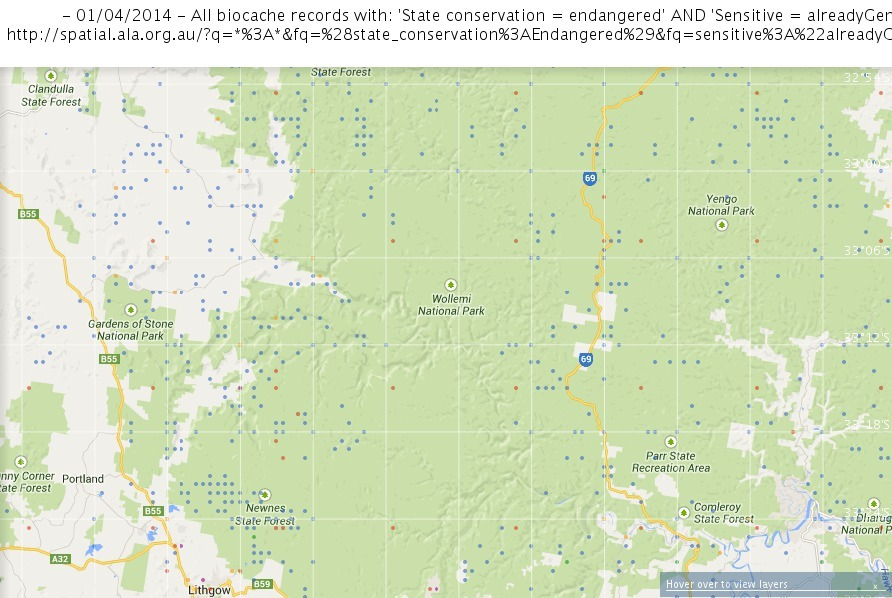# Introduction

2013/14 - i rescued this from the old sites portal, which is behind a closed door - maybe one day i'll revise it to read a little easier

This page sits within the broader context of spatial data, and applies to converting or obfuscating (denaturing / blurring) coordinate locations.

### For a primer on some important fundamentals of coordinate location data, see:

ALAhandlingspatiallocationdata.pdf

# Conversion

Depending on how data are presented, some (mathematical) conversion may be called for:

1. Darwincore would like decimal degrees.
2. ...
3. ...
4. ...

## Regarding co-ordinates in degrees ° (arc)minutes ' (arc)seconds " (DMS) vs. decimal degrees (DD)

these are two ways of displaying the same information - geographical (spatial) co-ordinates.

there is a mathematical relationship between the two the algorithm is as follows:

``````    decimal degrees = degrees + (minutes / 60) + (seconds / 3600)
``````

some considerations when performing this conversion:

1. be wary of floating point arithmetic and ambiguities: calculate once and store the value in a string to avoid inconsistent references to the same value; more info: http://www.validlab.com/goldberg/paper.pdf
2. if your smallest unit is minutes, truncate DD to three decimal places (hundreds of metres) to avoiding giving a false sense of precision; if seconds, truncate DD to five decimal places (metres)

## For conversion between geoco

There are a further two kinds of conversions to make on coordinate data:

1. converting between grid-coord's (cartesian - zone, eastings, northings) and geographic coord's (latitude/longitude pairs)
2. converting between one datum (eg, agd66) and another (currently gda94)

### Table of rough conversions

Measure Degrees Decimal degrees Metres (lat/long) (roughly, equator) Metres (lat) (roughly, poles) Metres (long) (roughly, poles)
One 1.00° 111,000 111,000 85,000
1' (arcmin) 1/60° 0.01666…° 1,700 1,700 1,300
1" (arcsec) 1/3600° 0.0002777…° 30 30 22
0.01° 1/100° 0.01° 1,000 1,000 760
0.001° 1/1000° 0.001° 100 100 76

Comparison between the different types of measurement - something to note: degrees of latitude are parallel so the distance between each degree remains almost constant, but since degrees of longitude are farthest apart at the equator and converge at the poles, their distance varies greatly.

# Obfuscation

Despite being a contentious topic there will always remain reasons for people to prefer recording a less precise location, especially as the tools for a more precise one become ever-nearer ubiquitous.

This section covers two methods for achieving this goal, truncating, and blurring.

Truncating is (potentially) a more robust method for denaturing/blurring locations, as its results are consistent.

If a randomisation is applied, the output result for a given input should be consistent, otherwise potential exists for statistical analysis of the result.

## Truncating

A simplified method of denaturing locations can be truncating the number of digits after the decimal point; here's a visual basic algorithm that will truncate lat/long's to 3 decimal places (~100m) -

`(fix(LAT_OR_LONG * 1000) / 1000)`

(note '^' is a placeholder for previous result)

1. `first, multiply the latitude by 1000 (FIELD * 1000)`
2. `next, discard anything decimal fix( ^ )`
3. `finally, return to 3 decimal places ( ^ ) / 1000`

in visual-basic's case, the 'fix' function converts a decimal to a whole number, and in the case of negatives, rounds upward toward 0; (this is in contrast to the 'int' function which does the same but rounds downward away from 0)

this method would also work in excel, but you would need to use the 'trunc' function (where a1 is the latitude):

`=( trunc( (a1*1000) ) / 1000 )`

these will wind up looking like a grid of dots on the map - here is an interesting example of this behaviour... note: if you click on the picture to load this dataset in the spatial portal, you'll need to zoom in on an area of interestAll biocache records with: 'State conservation = endangered' AND 'Sensitive = alreadyGeneralised'

## Blurring

For psuedo-code to convert + blur: GeospatialConversionPseudoCode.pdf - note: potentially flawed method of blurring referred to in this pdf link:

1. if a large number of sensitive records come from one point and are given a different (blurred) location each,
2. then statistically, the distribution of blurred locations around the point is of a smaller than expected area -
3. this could make it easier to determine the actual point shared by all records

A more complex, better distributed method of choosing random spots on a disc - http://mathworld.wolfram.com/DiskPointPicking.html